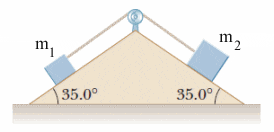# I have a hard time with kinematics involving inclined planes

## Homework Statement

Two blocks of mass m1 = 3.00 kg and m2 = 6.00 kg are connected by a massless string that passes over a frictionless pulley (see the figure below). The inclines are frictionless.

Image - https://www.webassign.net/serpse9/5-p-049.gifThe inclined plane is shaped like a triangle with both sides inclined at 35 degrees.

F = ma

## The Attempt at a Solution

For m1: Fnetx = ma ---> Fgx - T = ma ----> sin35(9.8m/s^2)(3kg) - T = 3kga
Equation for T: T = sin35(9.8m/s^2)(3kg) - 3kga

For m2: Fnetx = ma ---> Fgx - T = ma ----> sin35(9.8m/s^2)(6kg) - T = 6kga
Equation for T: T = sin35(9.8m/s^2)(6kg) - 6kga

Then I made them equal to each other in order to find acceleration but my answer ended up being wrong. Idk what I did wrong

#### Attachments

Last edited by a moderator:

kuruman
Homework Helper
Gold Member
2021 Award
You are not paying attention to your signs. For m1 the tension and the acceleration are in the same directon and for m2 the tension and the acceleration are in opposite directions. Assume the convention the anything uphill is positive and anything downhill is negative, i.e. the positive direction is the direction of the acceleration. Keeping the convention consistently, for m2 the acceleration is downhill, so anything downhill is positive and anything uphill is negative.

Chandra Prayaga